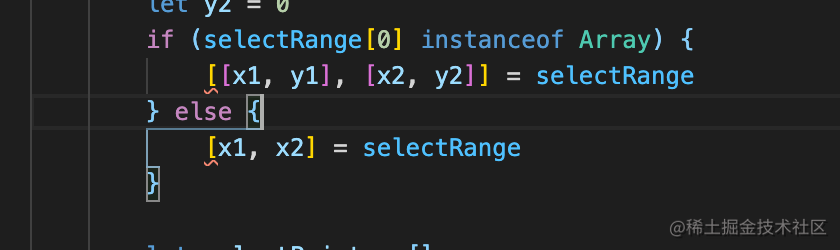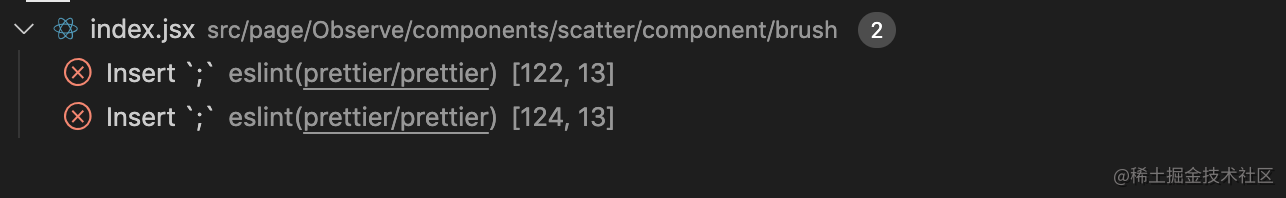# JS代码要不要加分号?

## 问题背景## 分号的作用

``````for(var i=1; i<=5; i++) {
console.log(i)
}
for(var i=1; i<=5; i++);{
console.log(i)
}

``````var a = 4
var b = [1,2,3]
[a]
console.log(b)

``````var a = 4
var b = [1,2,3][a]
console.log(b)

## 分号的添加规则

• 循环语句: `for`, `while`

• 分支语句: `if`, `switch`, `try`

• 函数声明 (不是函数表达式)

## ASI

### ASI的插入规则

• 以换行为基础。（就是你肯定得有换行符才行）

• JS解析器是尽量把语句合并成同一行的解析的。只有符合ASI规则的时候，才会加入分号进行断句。

#### 1、新行并入当前行将构成非法语句，自动插入分号。

``````if(true)
a = 1
console.log(a)
// 解析为
if(true) a = 1;
console.log(a);

``````if(true);
a = 1;
console.log(a);

#### 2、在continue, return, break, throw后自动插入分号。

``````return
123
// 解析为
return;
123;

#### 3、++、--后缀表达式作为新行的开始，在行首自动插入分号

``````var a = 1
var b = 2
a
++
b
// 解析成了
var a = 1
var b = 2
a;
++b;

#### 4、当出现一个不允许的行终止符或“}”时，会在其之前插入一个分号。

``````function(){ a = 1 }
// 解析成了
function(){ a = 1; }

## 个人公众号